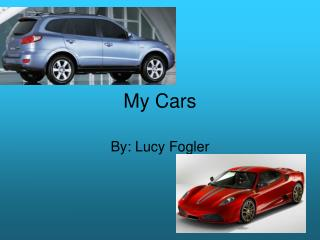DownloadDownload PresentationMy Cars

# My Cars

Télécharger la présentation## My Cars

- - - - - - - - - - - - - - - - - - - - - - - - - - - E N D - - - - - - - - - - - - - - - - - - - - - - - - - - -
##### Presentation Transcript

1. My Cars By: Lucy Fogler

2. New Car • 2008 Ferrari 599 GTB Fiorano Coupe F1 • \$273,845.00 • Interest rate: 4.99%* *courtesy of cefcu *courtesy of cefcu

3. Used Car • 2003 Hyundai SantaFe V6 • \$9,895.00 • Interest rate: 6.24%* *courtesy of cefcu

4. New Monthly Payment • Car is \$273,845.00 • Down payment of \$1000.00 • Car loan is \$272,845.00 • Monthly Payment Formula • R=P[(1-(1+i)^-n)/i]^-1 • When plugged into the formula the monthly payment is…. • \$5,147.67 P= 272,845 i= .0499/12 n= (12)(5)

5. Used Monthly Payments • Car is \$9,895.00 • Down Payment is \$1,000.00 • Car loan is \$8,895.00 • Monthly Payment Formula • R=P[(1-(1+i)^-n)/i]^-1 • When plugged into the formula the monthly payment is…. • \$172.96 P= 8,895 i= .0624/12 n= (12)(5)

6. Amortization Table

7. Amortization Explanation • Amount paid to interest • Used equation I=prt • P= (previous remaining balance) • r= (.0624) which is interest rate in decimal form • t= (1/12) one month is 1/12 of a year and t is in years • Amount paid to principal • Took monthly payment minus the amount paid to interest to get the amount paid to principal • Remaining balance • Took the previous remaining balance minus the amount paid to principal to get the new remaining balance • After 10 payments, I would still owe \$7,597.89 on my used car loan

8. New Car Used the formula: Total Interest=(monthly payment)(# of months paying)-amount borrowed Total Interest= (5147.67)(60)-272,845 Total Interest paid= \$36,015.20 Used Car Used the formula: Total Interest=(monthly payment)(# of months paying)-amount borrowed Total Interest= (172.96)(60)-8,895 Total Interest Paid= \$1,482.60 Total Interest

9. Affordability • I cannot afford the new car, but I can afford the used car. • I am not surprised because new car payments are usually more than \$300 if you are going to pay it off in only 5 years; however used cars are usually more affordable

10. Sources • New Car • http://www.autoweb.com/content/research/vir/index.cfm/vehicle_number_int/1025139/action/summary • Used Car • http://usedcars.kbb.com/fyc_b/vdp.jsp?ct=u&car_id=254868592&dealer_id=57948223&car_year=2003&model=SANTAFE&num_records=25&body_code=0&awsp=false&search_type=used&distance=25&search_lang=en&make=HYUND&default_sort=priceDESC&style_flag=1&LNX=KBBATVALFYCSRP&sort_type=priceDESC&address=61761&cardist=4 • Interest Rates • https://www.cefcu.com/about/rates.php#vehicle